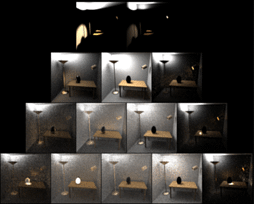## Optimally Combining Sampling Techniques for Monte Carlo Rendering

Reference: Eric Veach and Leonidas J. Guibas, SIGGRAPH 95 Proceedings (August 1995), Addison-Wesley, pp. 419-428.

Images too dark? Try the gamma corrected pages.
There is also more information on gamma correction.

### Abstract

Monte Carlo integration is a powerful technique for the evaluation of difficult integrals. Applications in rendering include distribution ray tracing, Monte Carlo path tracing, and form-factor computation for radiosity methods. In these cases variance can often be significantly reduced by drawing samples from several distributions, each designed to sample well some difficult aspect of the integrand. Normally this is done by explicitly partitioning the integration domain into regions that are sampled differently. We present a powerful alternative for constructing robust Monte Carlo estimators, by combining samples from several distributions in a way that is provably good. These estimators are unbiased, and can reduce variance significantly at little additional cost. We present experiments and measurements from several areas in rendering: calculation of glossy highlights from area light sources, the final gather'' pass of some radiosity algorithms, and direct solution of the rendering equation using bidirectional path tracing.

### Additional information

• Postscript of paper, minus color images and Figure 8 (490K)
• Postscript of full paper, with low-resolution grayscale images (2200K)
• Figure 2: Glossy highlights from area light sources
• (a) Sampling the light source (JPEG, 39K)
• (b) Sampling the BRDF (JPEG, 41K)
• (c) A weighted combination of samples from (a) and (b) using the power heuristic (JPEG, 34K)
• Figure 6: An area light source adjacent to a diffuse surface
• (a) Sampling the light source according to emitted power (JPEG, 15K)
• (b) Sampling the hemisphere according to projected solid angle (JPEG, 17K)
• (c) A weighted combination of samples from (a) and (b) using the power heuristic (JPEG, 14K)
• Figure 9: Bidirectional path tracing for global illumination
• (a) The weighted contribution that each bidirectional sampling technique makes to image (b) (JPEG, 197K)
• (b) Combines samples from all the bidirectional techniques shown in (a) using the power heuristic (JPEG, 53K, 25 samples per pixel)
• (c) Standard Monte Carlo path tracing using the same amount of work (JPEG, 92K, 56 samples per pixel)
If the images are too dark, try the gamma corrected pages. All JPEG images were compressed using a quality setting of 90.

### Extra images (in the electronic SIGGRAPH proceedings)

Figure 2(x) (JPEG, 22K)
A false-color image showing the weights used to compute Figure 2(c). Green represents samples from Figure 2(a), red from Figure 2(b). Yellow indicates that both types of samples are assigned a significant weight. Notice that some of the highlights are yellow in the center and green around the edges---the weights are not just a function of the light source size and surface roughness.

Figure 9(x) (JPEG, 312K)
This is an extension of Figure 9(a). Each image shows the weighted contribution made by one of the bidirectional sampling techniques to Figure 9(b), using the power heuristic with $\beta=2$. In this case, paths of up to length $k=6$ were sampled.

Row $i$ shows techniques that sample transport paths of length $i+1$. The $m$-th image in the $i$-th row uses the distribution $p_{i+1,m-1}$ described in Sec. 4.3 (this distribution chooses $m-1$ vertices using the light subpath, and the other $i+3-m$ vertices using the eye subpath). Images in row $i$ have been overexposed by $i$ f-stops so that details can be seen.

Relative to Figure 9(a), we have added one extra column to the left of each row (these images are nearly black, as mentioned in the text), and one row along the bottom (showing transport paths of length 6). We have still omitted one image from the right side of each row, corresponding to the sampling distribution $p_{i+1,i+2}$ (zero vertices in the light subpath). These images are truly black, since this particular scene has a pinhole lens.

Figure 9(y) (JPEG, 530K)
This image shows what the various bidirectional sampling techniques look like when used alone. This is similar to Fig. 2(a) and Fig. 2(b), where we used two different sampling techniques in attempting to compute the same image. In this case, the $m$-th image of row $i$ uses the distribution $p_{i+1,m-1}$ to make an image of the light flowing on paths of length $i+1$. Standard path tracing is equivalent to summing the second image from the left on each row (except for caustic paths, which get their contribution from the first image on each row).

Last modified: May 16, 1995
Eric Veach